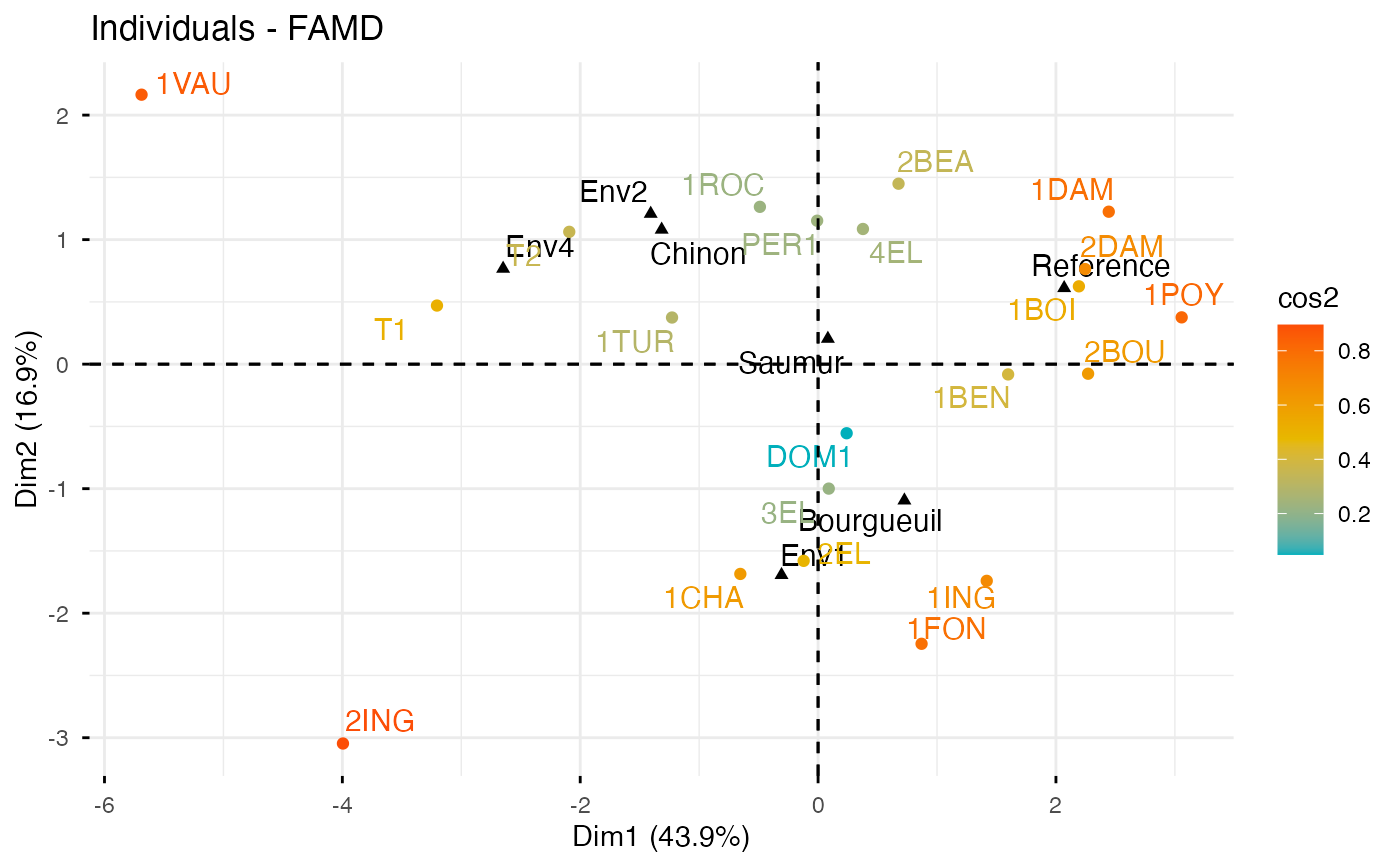Factor analysis of mixed data (FAMD) is, a particular case of MFA, used to analyze a data set containing both quantitative and qualitative variables. fviz_famd() provides ggplot2-based elegant visualization of FAMD outputs from the R function: FAMD [FactoMineR].

• fviz_famd_ind(): Graph of individuals

• fviz_famd_var(): Graph of variables

• fviz_famd(): An alias of fviz_famd_ind(res.famd)

fviz_famd_ind(X, axes = c(1, 2), geom = c("point", "text"),
repel = FALSE, habillage = "none", palette = NULL,
addEllipses = FALSE, col.ind = "blue", col.ind.sup = "darkblue",
alpha.ind = 1, shape.ind = 19, col.quali.var = "black",
select.ind = list(name = NULL, cos2 = NULL, contrib = NULL),

fviz_famd_var(X, choice = c("var", "quanti.var", "quali.var"),
axes = c(1, 2), geom = c("point", "text"), repel = FALSE,
col.var = "red", alpha.var = 1, shape.var = 17,
col.var.sup = "darkgreen", select.var = list(name = NULL, cos2 =
NULL, contrib = NULL), ...)

fviz_famd(X, ...)

## Arguments

X an object of class FAMD [FactoMineR]. a numeric vector of length 2 specifying the dimensions to be plotted. a text specifying the geometry to be used for the graph. Allowed values are the combination of c("point", "arrow", "text"). Use "point" (to show only points); "text" to show only labels; c("point", "text") or c("arrow", "text") to show arrows and texts. Using c("arrow", "text") is sensible only for the graph of variables. a boolean, whether to use ggrepel to avoid overplotting text labels or not. an optional factor variable for coloring the observations by groups. Default value is "none". If X is an MFA object from FactoMineR package, habillage can also specify the index of the factor variable in the data. the color palette to be used for coloring or filling by groups. Allowed values include "grey" for grey color palettes; brewer palettes e.g. "RdBu", "Blues", ...; or custom color palette e.g. c("blue", "red"); and scientific journal palettes from ggsci R package, e.g.: "npg", "aaas", "lancet", "jco", "ucscgb", "uchicago", "simpsons" and "rickandmorty". Can be also a numeric vector of length(groups); in this case a basic color palette is created using the function palette. logical value. If TRUE, draws ellipses around the individuals when habillage != "none". color for individuals and variables, respectively. Can be a continuous variable or a factor variable. Possible values include also : "cos2", "contrib", "coord", "x" or "y". In this case, the colors for individuals/variables are automatically controlled by their qualities ("cos2"), contributions ("contrib"), coordinates (x^2 + y^2 , "coord"), x values("x") or y values("y"). To use automatic coloring (by cos2, contrib, ....), make sure that habillage ="none". color for supplementary individuals controls the transparency of individuals and variables, respectively. The value can variate from 0 (total transparency) to 1 (no transparency). Default value is 1. Possible values include also : "cos2", "contrib", "coord", "x" or "y". In this case, the transparency for individual/variable colors are automatically controlled by their qualities ("cos2"), contributions ("contrib"), coordinates (x^2 + y^2 , "coord"), x values("x") or y values("y"). To use this, make sure that habillage ="none". point shapes of individuals, variables, groups and axes color for qualitative variables in fviz_mfa_ind(). Default is "black". a selection of individuals and variables to be drawn. Allowed values are NULL or a list containing the arguments name, cos2 or contrib: name is a character vector containing individuals/variables to be drawn cos2 if cos2 is in [0, 1], ex: 0.6, then individuals/variables with a cos2 > 0.6 are drawn. if cos2 > 1, ex: 5, then the top 5 individuals/variables with the highest cos2 are drawn. contrib if contrib > 1, ex: 5, then the top 5 individuals/variables with the highest cos2 are drawn vector of colors to use for n-colour gradient. Allowed values include brewer and ggsci color palettes. Arguments to be passed to the function fviz() The graph to plot inf fviz_mfa_var(). Allowed values include one of c("var", quanti.var", "quali.var"). color for supplementary variables.

a ggplot

## Examples

# Compute FAMD
library("FactoMineR")
data(wine)
res.famd <- FAMD(wine[,c(1,2, 16, 22, 29, 28, 30,31)], graph = FALSE)

# Eigenvalues/variances of dimensions
fviz_screeplot(res.famd)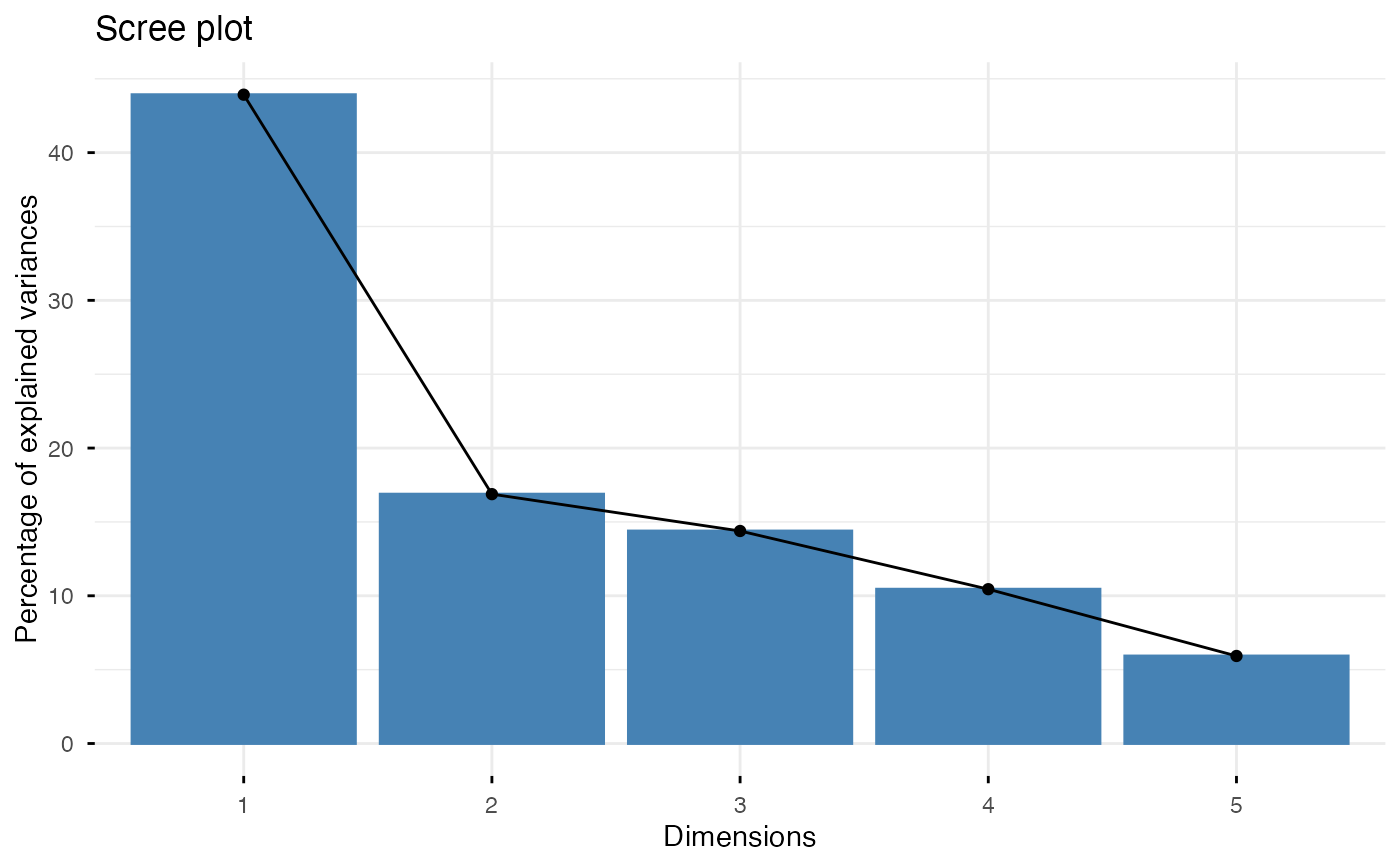# Graph of variables
fviz_famd_var(res.famd)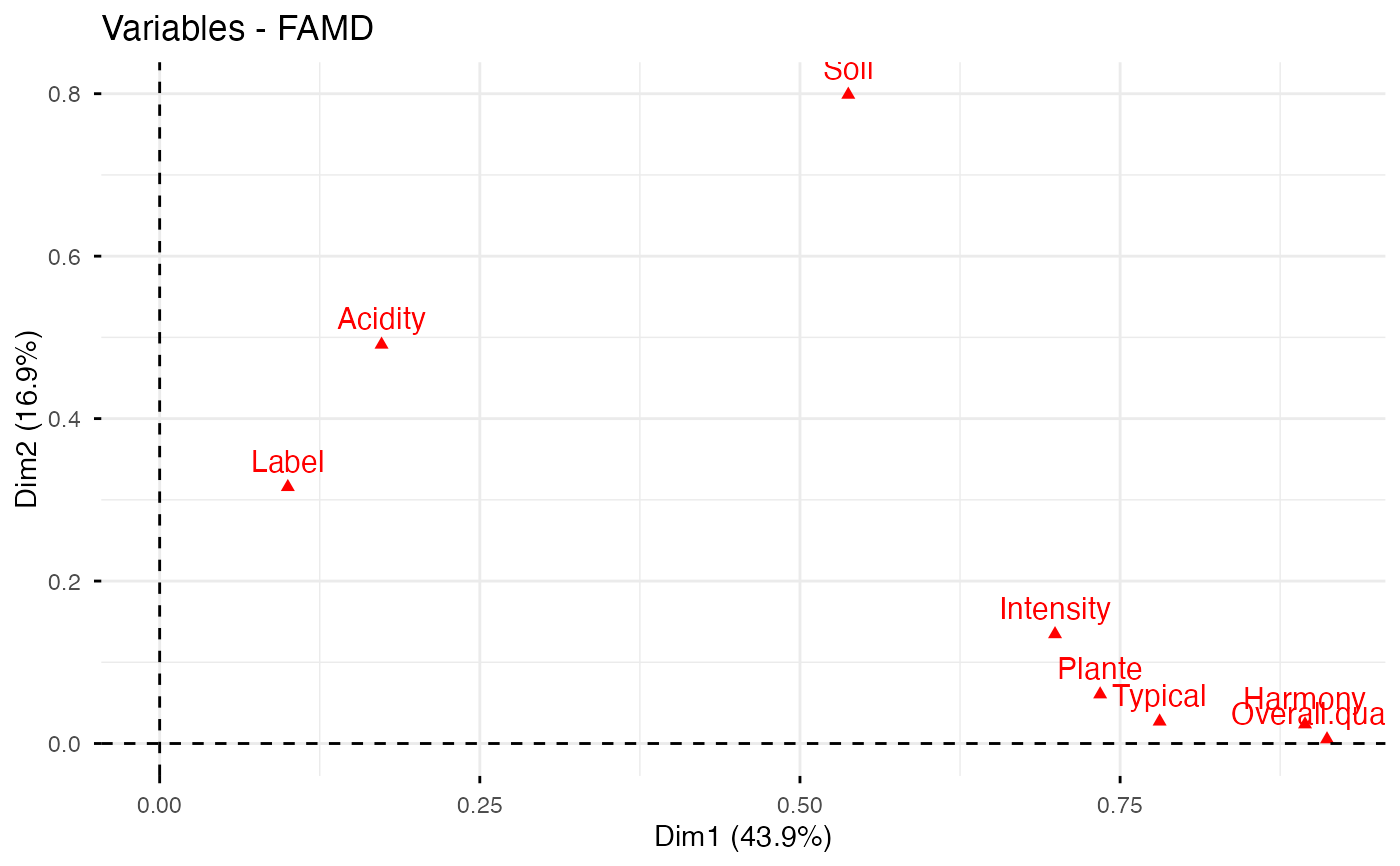# Quantitative variables
fviz_famd_var(res.famd, "quanti.var", repel = TRUE, col.var = "black")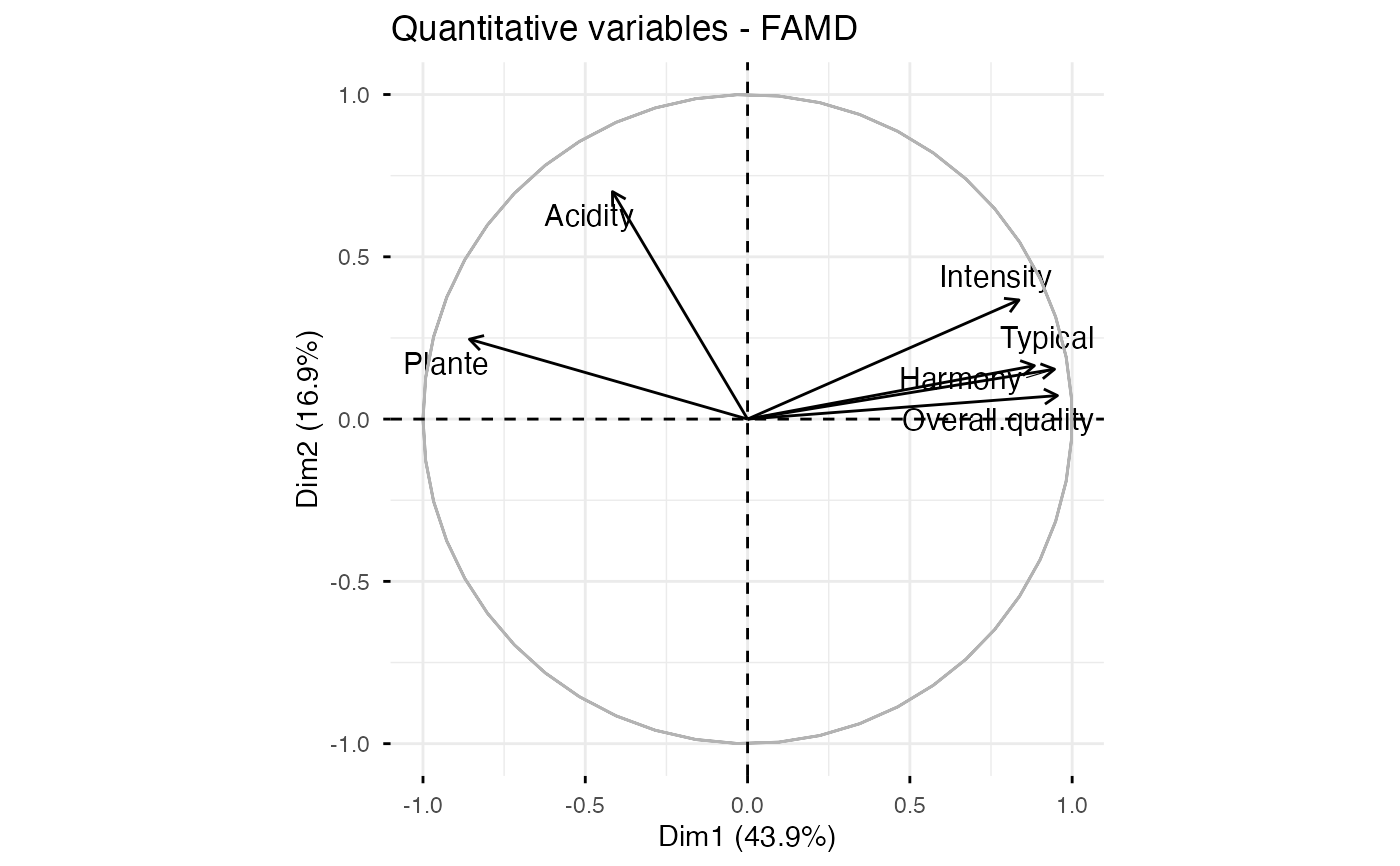# Qualitative variables
fviz_famd_var(res.famd, "quali.var", col.var = "black")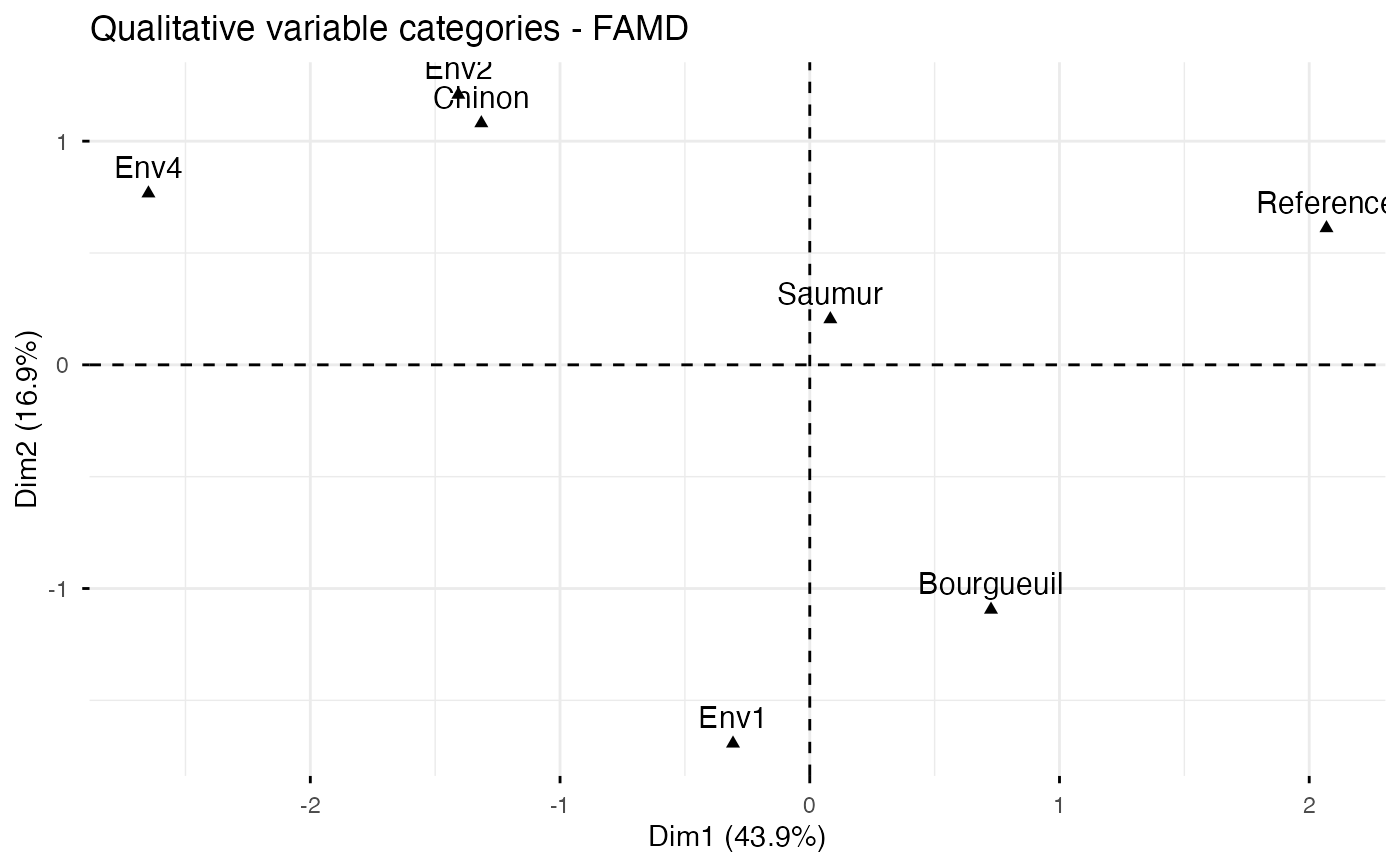# Graph of individuals colored by cos2
fviz_famd_ind(res.famd, col.ind = "cos2",
repel = TRUE)